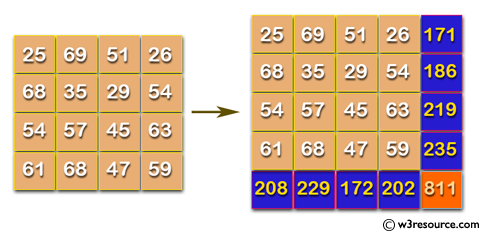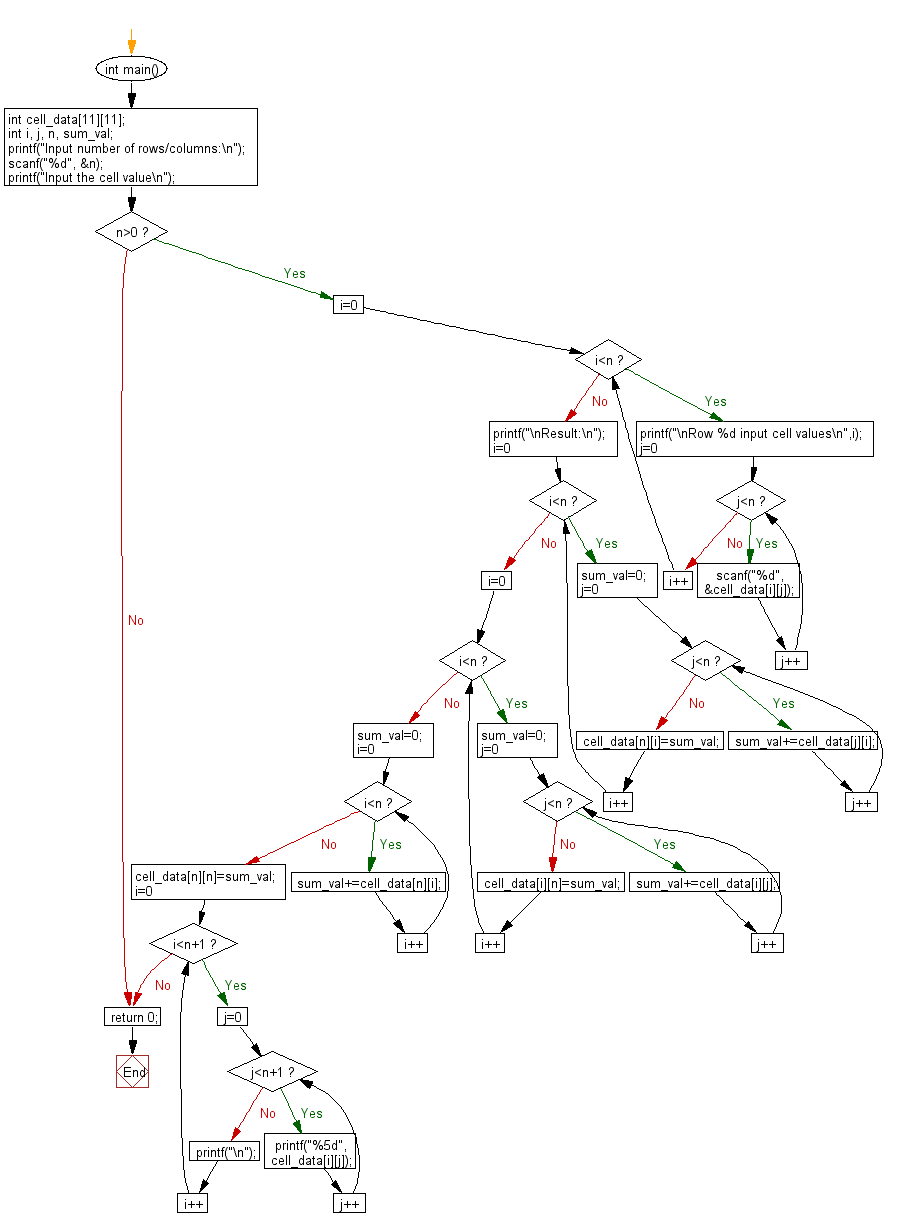﻿ C Program: Develop a small part of spreadsheet software - w3resource

# C Exercises: Develop a small part of spreadsheet software

## C Basic Declarations and Expressions: Exercise-148 with Solution

Write a C program, which adds up columns and rows of given table as shown in the following figure.Input:
n (the size of row and column of the given table)
1st row of the table
2nd row of the table
:
:
n th row of the table
The input ends with a line consisting of a single 0.
Output:
For each dataset, print the table with sum of rows and columns.

Sample Solution:

C Code:

``````#include <stdio.h>

int main() {
// Declare a 2D array to store cell data
int cell_data;
int i, j, n, sum_val;

// Prompt user for input
printf("Input number of rows/columns:\n");
scanf("%d", &n);

// Prompt user for cell values
printf("Input the cell value\n");

// Check if n is a positive value
if (n > 0) {
// Loop through rows
for(i = 0; i < n; i++) {
printf("\nRow %d input cell values\n", i);

// Loop through columns
for(j = 0; j < n; j++) {
// Read cell value and store it in the array
scanf("%d", &cell_data[i][j]);
}
}

// Calculate row sums and update array
printf("\nResult:\n");
for(i = 0; i < n; i++) {
sum_val = 0;
for(j = 0; j < n; j++) {
sum_val += cell_data[j][i];
}
cell_data[n][i] = sum_val;
}

// Calculate column sums and update array
for(i = 0; i < n; i++) {
sum_val = 0;
for(j = 0; j < n; j++) {
sum_val += cell_data[i][j];
}
cell_data[i][n] = sum_val;
}

// Calculate total sum and update array
sum_val = 0;
for(i = 0; i < n; i++) {
sum_val += cell_data[n][i];
}
cell_data[n][n] = sum_val;

// Print the updated array
for(i = 0; i < n + 1; i++) {
for(j = 0; j < n + 1; j++) {
printf("%5d", cell_data[i][j]);
}
printf("\n");
}
}

// Return 0 to indicate successful execution
return 0;
}
``````

Sample Output:

```Input number of rows/columns:
4
Input the cell value

Row 0 input cell values
25
69
51
26

Row 1 input cell values
68
35
29
54

Row 2 input cell values
54
57
45
63

Row 3 input cell values
61
68
47
59

Result:
25   69   51   26  171
68   35   29   54  186
54   57   45   63  219
61   68   47   59  235
208  229  172  202  811
```

Flowchart:C programming Code Editor:

What is the difficulty level of this exercise?

Test your Programming skills with w3resource's quiz.

﻿